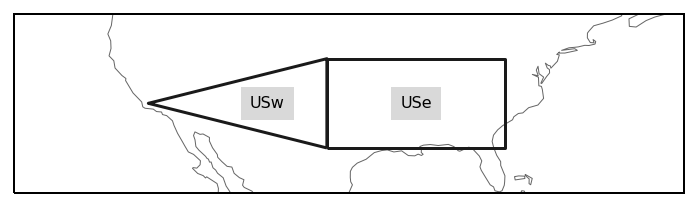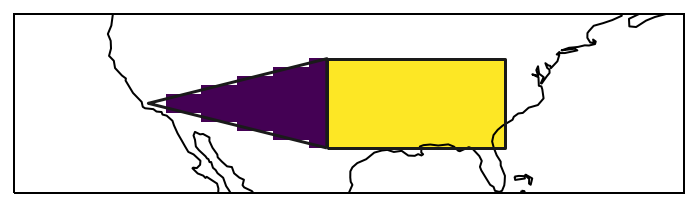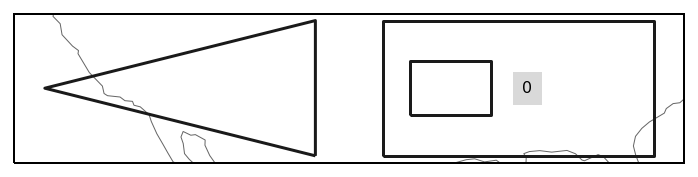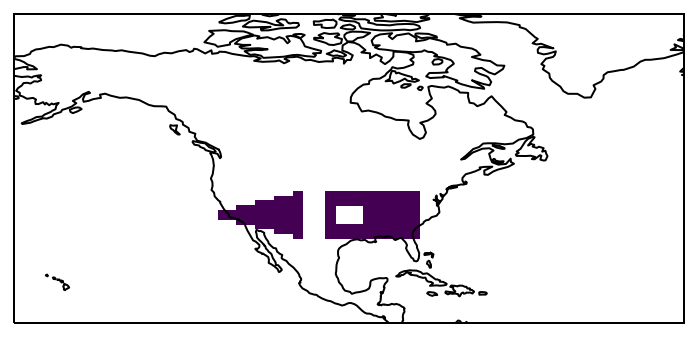Note

This tutorial was generated from an IPython notebook that can be downloaded here.

Note

In version 0.5 the `Regions_cls` has been renamed to `Regions` and it’s call signature has changed. All arguments (except `outlines`) are now optional.

Creating own regions is straightforward. Import regionmask and check the version:

```import regionmask

```
```'0.6.1'
```

Import numpy

```import numpy as np
```

Assume you have two custom regions in the US, you can easily use these to create `Regions`:

```US1 = np.array([[-100.0, 30], [-100, 40], [-120, 35]])
US2 = np.array([[-100.0, 30], [-80, 30], [-80, 40], [-100, 40]])

```
```<regionmask.Regions>
Name:     unnamed

Regions:
0  r0  Region0
1  r1  Region1

[2 regions]
```

If you want to set the `names` and `abbrevs` yourself you can still do that:

```names = ["US_west", "US_east"]
abbrevs = ["USw", "USe"]

USregions = regionmask.Regions([US1, US2], names=names, abbrevs=abbrevs, name="US")
USregions
```
```<regionmask.Regions>
Name:     US

Regions:
0  USw  US_west
1  USe  US_east

[2 regions]
```

Again we can plot the outline of the defined regions

```ax = USregions.plot(label="abbrev")

import cartopy.crs as ccrs

# fine tune the extent
ax.set_extent([225, 300, 25, 45], crs=ccrs.PlateCarree())
``````import numpy as np

# define lat/ lon grid
lon = np.arange(200.5, 330, 1)
lat = np.arange(74.5, 15, -1)

# for the plotting
lon_edges = np.arange(200, 331, 1)
lat_edges = np.arange(75, 14, -1)

```
```import matplotlib.pyplot as plt

ax = plt.subplot(111, projection=ccrs.PlateCarree())

# pcolormesh does not handle NaNs, requires masked array

h = ax.pcolormesh(
)

ax.coastlines()

# add the outlines of the regions

ax.set_extent([225, 300, 25, 45], crs=ccrs.PlateCarree())
```## Use shapely Polygon¶

If you have the region defined as a shapely polygon, this also works:

```from shapely.geometry import Polygon, MultiPolygon

US1_poly = Polygon(US1)
US2_poly = Polygon(US2)

US1_poly, US2_poly
```
```(<shapely.geometry.polygon.Polygon at 0x7f742a723590>,
<shapely.geometry.polygon.Polygon at 0x7f742a7231d0>)
```
```USregions_poly = regionmask.Regions([US1_poly, US2_poly])

USregions_poly
```
```<regionmask.Regions>
Name:     unnamed

Regions:
0  r0  Region0
1  r1  Region1

[2 regions]
```

## Create Regions with MultiPolygon and interiors¶

Create two discontiguous regions and combine them to one. Add a hole to one of the regions

```US1_shifted = US1 - (5, 0)
US2_hole = np.array([[-98.0, 33], [-92, 33], [-92, 37], [-98, 37], [-98.0, 33]])
```

Create `Polygons`, a `MultiPolygon`, and finally `Regions`

```US1_poly = Polygon(US1_shifted)
US2_poly = Polygon(US2, holes=[US2_hole])

US_multipoly = MultiPolygon([US1_poly, US2_poly])

```
```USregions_poly.plot();
``````mask = USregions_poly.mask(lon, lat)
```ax = plt.subplot(111, projection=ccrs.PlateCarree())